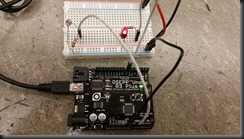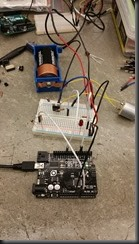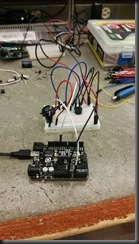# Part 10 – Micro-controllers, input and output

### Part 10 is where we learn to connect the hardware as both inputs and outputs using the code in the micro-controllers. This is the beginning of how to build bigger devices. The first part of Lab 10 is a primer on how to write some simple code to blink and LED connected to the Arduino. The Arduino setup looked like this….

 The first part of the code includes some compiler directives, next is the single pass setup routine that defines the line we’ll use on the Arduino and finally the loop code is where we blink the light. The code to do this looks like this: #define MYLED 7  // Our LED Pin #define LONGDELAY 16000 // A long delay void setup() { // put your setup code here, to run once: pinMode(MYLED,OUTPUT);  // Set LED pin for output }// Process our main LED blinking loop. void loop() { // put your main code here, to run repeatedly: digitalWrite(MYLED,HIGH); delayMicroseconds(LONGDELAY); // Tried microseconds digitalWrite(MYLED,LOW); delayMicroseconds(LONGDELAY); } The next part of Lab 10 includes learning about For loops and includes an example of first blinking the LED for a specific amount of time. The code to do that looks like this… #define MYLED 7  // Set our LED pin. #define LONGDELAY 1000 // Define a long delay #define SHORTDELAY 200 // Define a short delay void setup() { // put your setup code here, to run once: pinMode(MYLED,OUTPUT); // Initialize our LED pin   // Blink that LED 15 times using the counter   for (int counter=0;counter<15;counter++) { digitalWrite(MYLED,HIGH);  // Turn on the LED delay(SHORTDELAY); digitalWrite(MYLED,LOW);  // Turn off the LED delay(SHORTDELAY); } } // Use the longdelay in our main loop so we can see //   The difference void loop() { // put your main code here, to run repeatedly: digitalWrite(MYLED,HIGH); // Turn it on delay(LONGDELAY); digitalWrite(MYLED,LOW);  // Turn if off delay(LONGDELAY); } Next, we change the For loop characteristics to blink te LED in decreasing duration controlled by the For loop like this… #define MYLED 7 // The pin my LED will be connected to #define LONGDELAY 1000 // A long Delay #define SHORTDELAY 100 // A short delay void setup() { // put your setup code here, to run once: pinMode(MYLED,OUTPUT); // Setup the LED for output // Process through our for loop, note that we // Decrease our counter by 50 each time.  for (int counter=1000;counter>49;counter=counter-50) { digitalWrite(MYLED,HIGH);  // Turn on the LED delay(counter); digitalWrite(MYLED,LOW); delay(counter); } }// Process our main program loop that will just blink //   the LED void loop() { // put your main code here, to run repeatedly: digitalWrite(MYLED,HIGH); delay(LONGDELAY); digitalWrite(MYLED,LOW); delay(LONGDELAY); } Next in the lab, since until this point we’ve only been controlling output, we attached a simple switch to our board and wired it to a pin to use as an input.#define MYLED 7  // Define LED Pin #define MYBUTTON 10 // Define button pin int buttonState = LOW;  // Create a global variable for the button state // Using LOW for off and HIGH for on. void setup() { // put your setup code here, to run once: pinMode(MYLED,OUTPUT); // Initialize the LED output pinMode(MYBUTTON,INPUT); // Initialize the button for input digitalWrite(MYLED,LOW); // Make sure the LED is turned off } void loop() { // put your main code here, to run repeatedly: // First we need to read what the button is doing. buttonState = digitalRead(MYBUTTON); // Check if button pressed if (buttonState == HIGH) { digitalWrite(MYLED,HIGH); } else // if (buttonState == LOW) { digitalWrite(MYLED,LOW); } } So now we have a button that is using software to control the LED. Lots of possibilities now that we can control input and output. We learned a little more about comparison operators and code flow. Next, the rest of the lab is showing how to use different types of inputs and outputs. We start by replacing the simple button switch we just used with a Light Detecting Resistor (LDR).#define MYLED 7 #define MYSENSOR A0 int sensorvalue = 0; void setup() { // put your setup code here, to run once: pinMode(MYLED,OUTPUT); // Initialize the LED output digitalWrite(MYLED,LOW); // Make sure the LED is turned off } void loop() { // put your main code here, to run repeatedly: sensorvalue = analogRead(MYSENSOR); // Read the current sensor value if (sensorvalue>750) { digitalWrite(MYLED,HIGH); // Turn on the LED delay(sensorvalue); } else { digitalWrite(MYLED,LOW); // Turn off the LED delay(sensorvalue); }} [amazon_link asins=’B01D8KOZF4,B01EWNUUUA,B01CZTLHGE,B01EWOE0UU’ template=’ProductGrid’ store=’scottkerfootcom-20′ marketplace=’US’ link_id=’e52c9209-9a98-11e8-b664-77ea3925fbdd’] Next, we control 2 different LED’s based on how much light is going into the LDR. Special note: At this point I used a bit of my past experience with coding to create a little bit of code that will automatically learn the min and max of the resistor that was connected. I figured this code would be useful for the rest of the lab, I was right. Here is the LDR.  The code below controls both the two LED’s and the motor. #define MYLED1 7 // The first LED #define MYLED2 8 // The second LED #define MYSENSOR A0 // The LDR Sensor pin #define MYMOTOR 11 // My motor pin int sensorvalue = 0; // Global for sensor value. float sensorvaluefloat = 0; // Sensor calculation float sensormin = 1024; // Sensor mininum value float sensormax = 0; // Sensor maximum value float sensorrange = 0; // Total range for the sensor float sensorpos = 0; // This will be our sensor position based on the range we learn float sensoron1 = 0; float sensoron2 = 0; float sensoron3 = 0; float motorcalc = 0; int motorspeed = 0; int lastmotorspeed = 0; void setup() { // put your setup code here, to run once: pinMode(MYLED1,OUTPUT); // Initialize the LED output pinMode(MYLED2,OUTPUT); // Initialize the second LED digitalWrite(MYLED1,LOW); // Make sure the LED is turned off digitalWrite(MYLED2,LOW); // Make sure the other LED is turned off // Setup our debug ability Serial.begin(9600); } void loop() { // put your main code here, to run repeatedly:   // First we need to read from our sensor sensorvalue = analogRead(MYSENSOR); // Read the current sensor value Serial.print(sensorvalue); Serial.print(” “); // Serial.println(sensorvalue); // For debugging purposes // Then we need to learn the upper and lower bounds of the sensor.   // Create a float version of sensorvalue sensorvaluefloat = float(sensorvalue);if (sensorvaluesensormax) sensormax = sensorvalue; Serial.print(sensormax); Serial.print(” “); // now we decide where in the sensor range we land. sensorrange = sensormax – sensormin; Serial.print(sensorrange); Serial.print(” “); // Max for range 1 sensoron1 = sensormin + (0.35 * sensorrange); // Max for range 2 sensoron2 = sensormin + (0.60 * sensorrange); // Max for range 3 sensoron3 = sensormin + (0.80 * sensorrange);   motorspeed = 255; // Light up the LED’s based on where we land. if (sensorvaluesensormax) sensormax = sensorvalue; Serial.print(“Max-“); Serial.print(sensormax); Serial.print(” “); // now we decide where in the sensor range we land. sensorrange = sensormax – sensormin; Serial.print(“Rng-“); Serial.print(sensorrange); Serial.print(” “);   // Now we need to run the motor motorcalc = ((sensorvalue – sensormin)/sensorrange) * MAXWRITE; Serial.print(“Spc-“); Serial.print(motorcalc);   // Create the motor speed motorspeed = int(motorcalc);   if (motorspeed < 150) { motorspeed = 0; } else { motorspeed =255; } // Print the speed assigned Serial.print(“Spd-“); Serial.print(motorspeed); Serial.print(” “);   // Light up the LED with the corresponding motor speed brightness analogWrite(MYLED1,motorspeed);   // Set the motor speed analogWrite(MYMOTOR,motorspeed);lastmotorspeed = motorcalc;   delay(100); Serial.println(” “); }Next we implemented a buzzer controlled by a  potentiometer. #define MYPOT A0 #define MYBUZZER 11 #define OUTPUTMAX 255 int sensorvalue = 0; float sensormin = OUTPUTMAX; float sensormax = 0; float sensorcalc = 0; float sensorrange = 0; float outputcalc = 0; int outputval = 0; void setup() { // put your setup code here, to run once: pinMode(MYPOT,INPUT); pinMode(MYBUZZER,OUTPUT); // Initialize the POT for input // Setup our debug ability Serial.begin(9600); } void loop() { // put your main code here, to run repeatedly:   // First we need to read from our sensor sensorvalue = analogRead(MYPOT); // Read the current sensor value Serial.print(sensorvalue); Serial.print(” “);   sensorcalc = float(sensorvalue); // cast the sensorvalue to a float Serial.print(sensorcalc); Serial.print(” “);if (sensormin>sensorcalc) sensormin = sensorcalc; // Assign the min for the sensor values Serial.print(sensormin); Serial.print(” “);   if (sensormax0) buzzme();Serial.println(” “); delay(20); }Part 10, a long one, complete!

This site uses Akismet to reduce spam. Learn how your comment data is processed.